Units of measurements  by  Charles Monari

6

### MILLIMETRE

Millimetre is a tenth of a centimetre.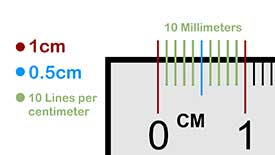Millimetre and centimetre

There are 10 millimetres in 1 centimetre

∴ 10 mm = 1 cm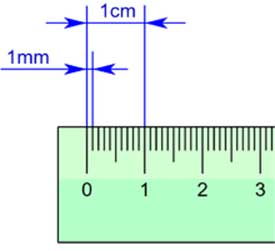Millimetre is the smallest unit of measurement

1 cm=10mm

1dm=100mm

1M  = 1000mm

1Dm=10000mm

1Hm=100000mm

1Km=1000000mm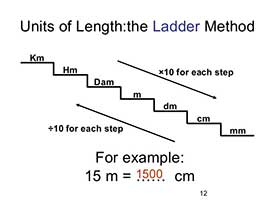• Converting millimetre into centimetre

1 cm = 10 mm

40mm = 40 ÷ 10 = 4 cm

6 cm = 6 x 10 = 60 mm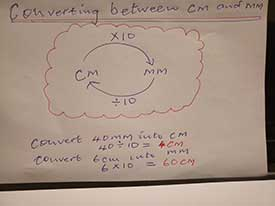• Converting between cm and mm

Convert millimeters to centimeters

Example 1:

80 mm = _____ cm?

80 mm = 80 mm ÷ 10 mm

= 8 cm

Example 2:

130 mm = _____ cm

130 mm = 130 mm ÷ 10 mm

= 13 cm

Convert centimeters to millimeters

Example 1:

55 cm = _____ mm?

55 cm = 55  x 10 mm

= 550 mm

Example 2:

7.8 cm = _____ mm

7.8 cm = 7.8 x 10 mm

= 78 mm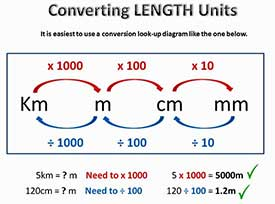Question

Convert the following measurements to the units indicated:

(a) 7 cm to mm

(b) 8 m to cm

(c) 9 km to m

Solution: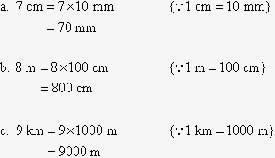Convert the following measurements to the units indicated:

(a) 6 m to mm (b)  4 km to mm

1 m    = 1000mm

6 m   = 6 x 1000

= 6000mm

1 Km = 1000 000mm

4 Km   = 4 x 1000 000

= 4 000 000 mm

How many millimetres are the in

(a) 41cm 6mm  (b) 74 cm 3mm

(a) 41cm 6mm

41cm= 41cm x 10

=410 mm

Total  = 410mm + 6mm

= 416 mm

(b) 74cm 3mm

74cm = 74cm x 10

=740mm

Total=740mm+3mm

=743mm

Brians’ string is 1m 85cm. What is the length in millimetres?

1m 85 cm

1m  = 1m x 1000

= 1000mm

85cm  = 85cm x 10

= 850mm

Total    = 1000mm + 850mm

= 1850mm

∴ Brians’ string is 1850mm

Questions

How many millimetres are there in

(a) 2m 36cm 7mm?
(b) 7m 91cm 4mm?

Questions

How many millimetres are there in (a) 2m 36cm 7mm? (b) 7m 91cm 4mm?

Solution

2m 36cm 7mm

2m = 2m x 1000

=2000mm

36cm= 36cm x 10

=360mm

7mm = 7mm

Total = 2000mm+360mm+7mm

= 2360 mm

solution

7m 91cm 4mm

7m= 7m x 1000

=7000mm

91cm= 91cm x 10

=910mm

4mm = 4mm

Total = 7000mm+910mm+4mm

= 7910 mm